# AP Chemistry : Interpreting Graphs and Tables

## Example Questions

### Example Question #1 : Interpreting Graphs And Tables

If a solution of water has a pressure of 200atm and a temperature of 474 degrees Celsius, what changes could be made to bring the sample to its critical point?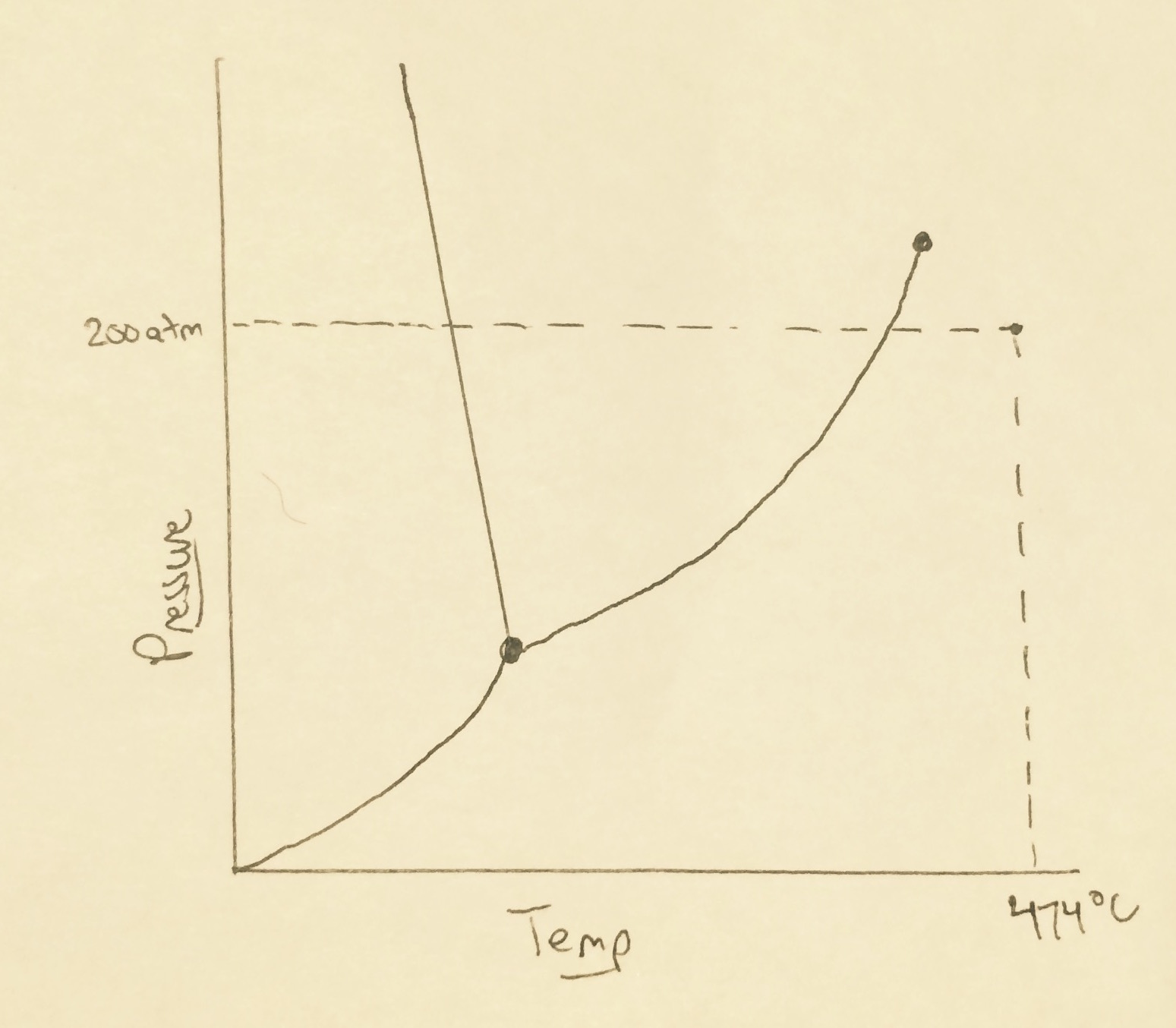Decrease the pressure and decrease the temperature

Increase the pressure and decrease the temperature

The critical point cannot be reached from these starting conditions

Increase the pressure and increase the temperature

Decrease the pressure and increase the temperature

Increase the pressure and decrease the temperature

Explanation:

The critical point for water is at 218atm and 374 degrees Celcius, but on this graph it can be found at the end of the line between the gas and liquid portions of the water phase graph (you can see it end with a dot). This means we need to increase our pressure and decrease our temperature in order to reach the critical point from our initial conditions.

### Example Question #2 : Interpreting Graphs And Tables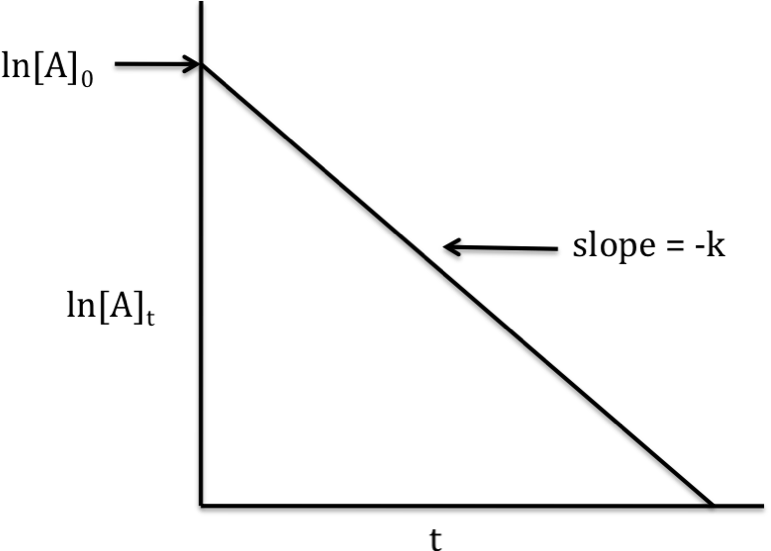A chemistry student is trying to identify the reaction order for a reaction based on her interpretation of the above graph. The student identifies the reaction as a first-order reaction. Is this student correct in their conclusion? Why?

No. A graph of the natural logarithm of the concentration of a reactant versus time yields a straight line with a negative slope for a zeroth-order reaction.

No. A graph of the natural logarithm of the concentration of a reactant versus time yields a straight line with a negative slope for a second-order reaction.

Yes. Regardless of the order of the reaction, a graph of the natural logarithm of the concentration of a reactant versus time will always yield a straight line with a negative slope.

Yes. A graph of the natural logarithm of the concentration of a reactant versus time yields a straight line with a negative slope for a first-order reaction.

Additional information is necessary in order to determine whether the graph corresponds to a first-order reaction.

Yes. A graph of the natural logarithm of the concentration of a reactant versus time yields a straight line with a negative slope for a first-order reaction.

Explanation:

A first-order reaction can be expressed in different ways. One way is the following: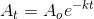where: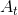= concentration of reactant at a given time,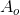= initial concentration of reactant at time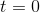= rate constant of the reaction= amount of time that has passed since the start of the reaction

By taking the natural logarithm of both sides, we can obtain an alternative form of the equation, expressed as: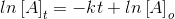This second equation is useful for graphing, because it fits the general equation of. In this case,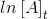represents the-axis,represents the-axis,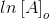represents the-intercept, and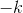represents the slope. Thus, the graph in the question stem is indeed indicative of a first-order reaction.

In the case of a zeroth-order reaction, the equation is: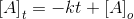Therefore, for a zeroth-order reaction, a straight line with a negative slope will only result from a graph of the concentration of a reactant versus time.

And lastly, in the case of a second-order reaction, the equation is: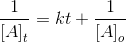Hence, a graph of the inverse concentration of a reactant versus time will yield a straight line with a positive slope, and this is indicative of a second-order reaction.